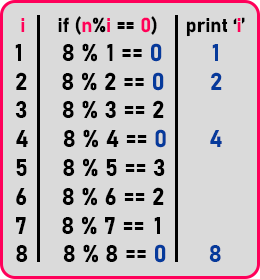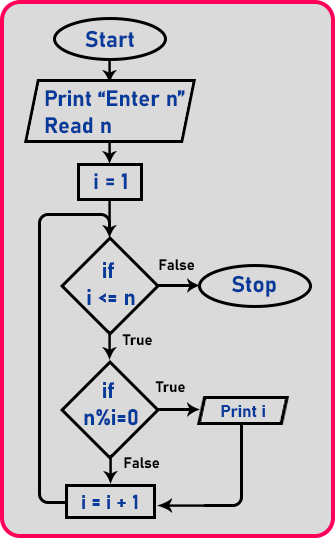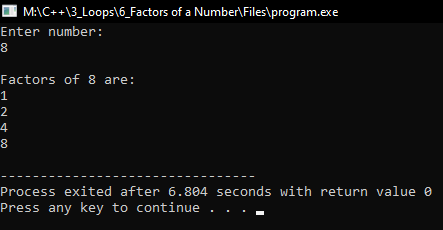# Factors of a Number using Loop in C++

## Factors of a Number using Loop in C++

In this article, I am going to discuss Program to Print Factors of a Number using Loop in C++ with Examples. Please read our previous articles, where we discussed the Factorial of a Number using Loop in C++ with Examples.

##### Factors of a Number:

First, we will explain what is meant by a factor. Then we’ll see the procedure and then a flowchart and program. Let’s take a number ‘n = 8’ and now we will find the factors of 8. If we divide ‘8’ by some number it is exactly getting divided or the remainder is ‘0’ then it is called a Factor.

Now, who can be the factors? Factors can be from ‘1’ to ‘8’. Some numbers from ‘1’ to ‘8’ can be the factors of ‘8’. Factors are ‘1’, ‘2’, ‘4’ and ‘8’. Non-factors are ‘3’, ‘5’, ‘6’ and ‘7’.

We have already discussed the modulo operator in the arithmetic operation article. Modulo operator is used to getting the remainder of the division between two numbers. Here we will use the modulo operator. Those will be the factors whose modulo will be ‘0’ and the number whose modulo is other than ‘0’ then that will not a factor of that number.

So, if the mod will be zero then we will print ‘it is a factor’ and if the mod is not zero then we will print ‘it is not a factor’. Now let us do some paperwork to explain in a better way.In the above table, the left-hand side column is for counter ‘i’ means we have to check factors from 1 to that number which we want the factors, in this case, ‘8’, so from ‘1’ to ‘8’. The mid column will check for the condition, ‘if (n % i == 0)’, here we are checking which number will exactly divide ‘8’. For exact division, the remainder must be 0.

So, in the right-hand side column, we are printing the numbers that exactly divide ‘8’ or leaving the remainder ‘0’. So, we will print ‘1’, ‘2’ ‘4’, and ‘8’ are the factors of ‘8’. Now let us look at the flowchart.

##### Factors of a Number Flowchart:Let us explain the flowchart. First, we will take a number from the user, it is the number for which we want to find the factors. Next, we will initialize our counter, in this case, it is ‘i’. We will assign ‘i’ to 1. Next, we will check for the condition ‘if (i <= n)’, it is the condition to run our loop. If it is false then exit the loop.

Now, if the condition ‘if (i <= n)’ will true then we will check for another condition which is ‘if (n % i == 0)’, this condition will check for, will counter ‘i’ number give 0 remainders on division with the given number.

If the condition is true, means the number is exactly divisible by another number then print that number means print ‘i’. If it is false then increment the counter and again control goes to the first condition and it will continue until the loop condition ‘if (i <= n)’ fails. So, this was the procedure to find the factors for the given number. Now let us look at the program.

##### Program to find the factors of given number using loop in C++:
```#include <iostream>
using namespace std;
int main()
{
int n;
cout << "Enter number: " << endl;
cin >> n;
cout << endl;
cout << "Factors of " << n << " are:" << endl;
for (int i = 1; i <= n; i++)
{
if (n % i == 0)
{
cout << i << endl;
}
}
return 0;
}
```
###### Output: